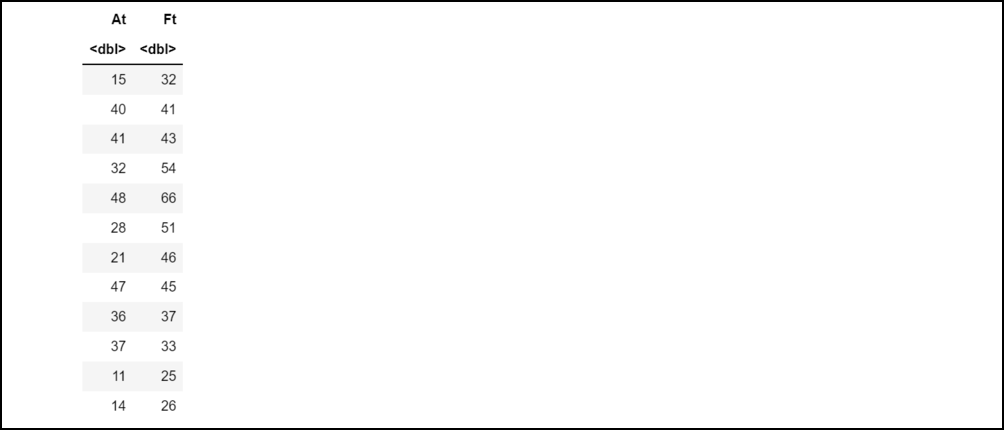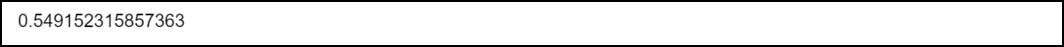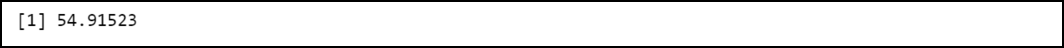# How to Calculate MAPE in R

• Last Updated : 25 Apr, 2022

In this article, we are going to see how to calculate MAPE in R Programming Language.

## MAPE:

It is an acronym for mean absolute percentage error. MAPE is used to measure the accuracy of a forecast system. The accuracy is measured in terms of percentage. MAPE can be calculated using the following formula:

```MAPE = (1 / n) * Σ(|At – Ft| / |At|) * 100

Here,
n: Represents the number of fitted points
At: Represents the actual value,
Ft: Represents the forecast value.
Σ: Symbol used to denote summation```

MAPE is quite common and used to forecast errors. It works most efficiently when their extremes don’t exist in the data.

## Method 1: Using MLmetrics package

In R, the MLmetrics package provides us the MAPE() function using which we can calculate the MAPE in R. This function has the following syntax:

Syntax: MAPE(Ft, At)

Parameters:

Here,

• Ft: It represent forecasted values
• At: It represents actual values

Example:

## R

 `# Create a dataframe` `dataframe <- ``data.frame``(At=``c``(15, 40, 41, 32, ` `                             ``48, 28, 21, 47, 36,` `                             ``37, 11, 14),` `                   ``Ft=``c``(32, 41, 43, 54, 66, 51, ` `                        ``46, 45, 37, 33, 25, 26))`   `# Print the dataframe` `dataframe`

Output:Now to find out the MAPE of the above data frame, we can use MAPE() function:

## R

 `# Import library` `library``(``"MLmetrics"``)`   `# Create a dataframe` `dataframe <- ``data.frame``(At=``c``(15, 40, 41, 32,` `                             ``48, 28, 21, 47,` `                             ``36, 37, 11, 14),` `                   ``Ft=``c``(32, 41, 43, 54, 66, ` `                        ``51, 46, 45, 37, 33, ` `                        ``25, 26))`   `# Compute MAPE` `MAPE``(dataframe\$Ft, dataframe\$At)`

Output:Hence, the MAPE value comes out to be equal to 54.915 %.

## Method 2: Creating a custom function

We can create our own function to determine MAPE.

Example:

Let us firstly create a data frame that contains two columns. One column holds actual values and the other column holds forecasted values.

## R

 `# Create a dataframe` `dataframe <- ``data.frame``(At=``c``(15, 40, 41, 32, 48, ` `                             ``28, 21, 47, 36, 37,` `                             ``11, 14),` `                   ``Ft=``c``(32, 41, 43, 54, 66, 51, ` `                        ``46, 45, 37, 33, 25, 26))`   `# Print the dataframe` `dataframe`

Output:Now we need to create a function that computes MAPE for the above-created data frame:

Note that  (1 / n) * Σ(|At – Ft| / |At|) is equivalent to the mean value of :

`abs(( dataframe\$At-dataframe\$Ft) / dataframe\$At)`

Thus we can mean() function in R. This function has the following syntax:

Syntax: mean(vect, na.rm)

Parameters:

• vect: It represents the numeric vector
• na.rm: Boolean value to ignore NA value

Now we can multiply this value by 100 and return the final value from the function.

## R

 `# Custom function that computes` `# MAPE of the passed dataframe` `calculateMAPE <- ``function``(dataframe) {` `        `  `        ``result = ``mean``(``abs``((dataframe\$At-dataframe\$Ft)` `                          ``/dataframe\$At)) * 100` `        ``return``(result)` `}`   `# Create a dataframe` `dataframe <- ``data.frame``(At=``c``(15, 40, 41, 32, ` `                             ``48, 28, 21, 47, ` `                             ``36, 37, 11, 14),` `                   ``Ft=``c``(32, 41, 43, 54, 66,` `                        ``51, 46, 45, 37, 33,` `                        ``25, 26))`   `# calculate MAPE` `result = ``calculateMAPE``(dataframe)`   `# Print the result` `print``(result)`

Output:Hence, the MAPE value comes out to be equal to 54.915 %.

My Personal Notes arrow_drop_up
Recommended Articles
Page :